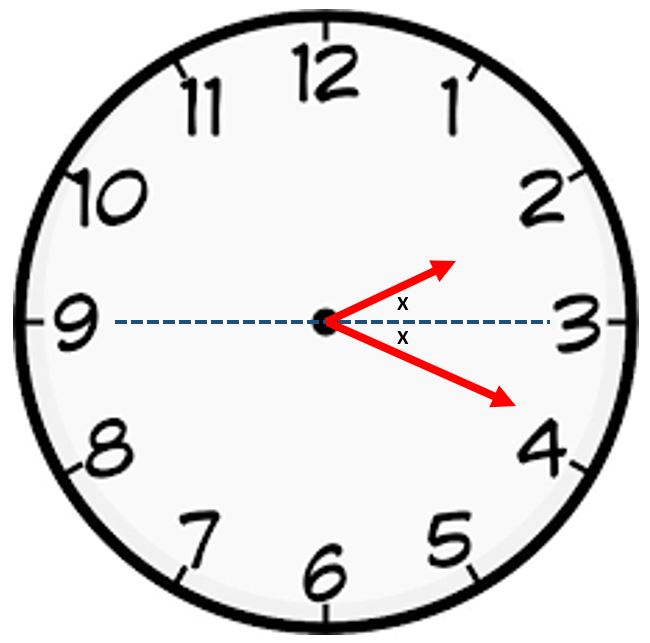# Symmetric TimeAt some time close to a quarter past two, the hour and minute hands of the clock are mirror images of each other, with respect to the horizontal line through 3 o'clock.

What is the precise time that this happens? Round off to the nearest second; give the answer in the form $\text{HMMSS}$. (For instance, if the answer were 2:12:41, type 21241.)

Extra challenge: How many times does a symmetric situation like this occur in a 12-hour period?

×